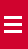# What is solid height?

Solid height of a compression spring is the point at which all its coils touch each other, and no more force can be exerted by the spring. Solid height is a key factor involved in the design of compression springs.

A compression spring may exert great force, but solid height can reduce those numbers. One of the most common examples of a compression spring attempting solid height is a ball point pen. When a button at the pen end is activated, the compression springs travels to the solid height, and exerts the force required to push the tip, which protrudes out of the pen. When the pen is pushed again, the spring is released, and it returns to its earlier state retracting the tip of the pen into the body.

### How to calculate solid height?

To calculate the solid height, you need to consider the types of ends on your spring. One of the most economical types of compression ends are squared and closed ends. If you need the spring to lay flat on a surface without a wobble effect of the regular squared and closed ends, then ground and closed ends are ideal. However, the grounding will add to your costs, therefore it is important to ensure its necessity.

If the ends of the spring need to be threaded, then it is ideal to buy open ended compression spring. If your design demands threading the spring onto a device or requires it to stay stable at the bottom and top, then multiple closed ends, also known as double closed ends are ideal.

Here is how you can calculate the solid heights of all variants discussed above:

• For Closed and Squared Ends

Total Wires = Total Coils + 1

Total Wire x Wire Diameter = Solid Height

• For Closed and Ground Ends

Total Wires = Total Coils

Total Wire x Wire Diameter = Solid Height

• For Double Closed Ends

Total Coils + 1 Wire = Total Wires

Total Wires x Wire Diameter = Solid Height

• For Open Ends

Total Coils + 1 Wire = Total Wires

Total Wires x Wire Diameter = Solid Height